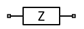Documentation

# Z (Impedance)

Model complex impedance

## Library

Elements## Description

The Impedance block implements the relation, vk(t) = Z(fk)*ik(t), for each simulation frequency, fk, where:

• Z(fk) represents complex-valued impedance at a specified simulation frequency.

• vk(t) represents the voltage across the terminals of the element at time t.

• ik(t) represents the current through the element at time t.

Circuit envelope current and voltage signals comprise in-phase, Ik, and quadrature, Qk, components at each frequency, fk.

Frequency-dependent impedance typically cannot be realized as a physical network such as an RLC chain. You can, however, use the Impedance block to model nonphysical behavior, such as frequency-independent negative capacitance or negative inductance. You can also use this block to specify resonant frequency offsets in filter networks.

## Parameters

Impedance type

Choose `Frequency independent` to apply the same impedance for all frequencies. Choose ```Frequency dependent``` to apply the impedance as a piecewise linear function.

Complex impedance (ohm)

When Impedance type is set to ```Frequency independent```, impedance is a scalar complex number that is applied to all simulation frequencies. When Impedance type is set to ```Frequency dependent```, impedance is a vector of complex numbers, [Z1, Z2, Z3, …]. This vector is linearly interpolated for all simulation frequencies.

In both cases, for zero simulation frequency, the imaginary part of the impedance is ignored. Also, the real part is forced to be positive to produce a stable simulation.

Frequency

When Impedance type is set to ```Frequency dependent```, specify a vector of nonnegative frequencies, [f1, f2, f3, …]. The pairs, fi, Zi, define a piecewise linear function, Z(f), that is linearly interpolated for the simulation frequencies. For values outside the range, there is constant extrapolation. The default value of this parameter is `0` Hz.

## Examples

The example, Frequency Response of an RF Transmit/Receive Duplex Filter, simulates an analog RF filter comprising Capacitor, Inductor, Resistor, and Impedance blocks.